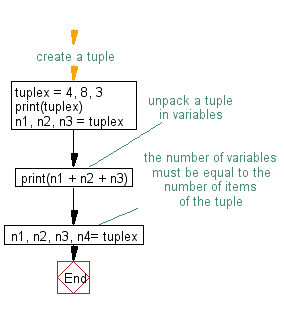﻿ Python: Unpack a tuple in several variables - w3resource# Python Exercise: Unpack a tuple in several variables

## Python tuple: Exercise-4 with Solution

Write a Python program to unpack a tuple in several variables.

Sample Solution:-

Python Code:

``````#create a tuple
tuplex = 4, 8, 3
print(tuplex)
n1, n2, n3 = tuplex
#unpack a tuple in variables
print(n1 + n2 + n3)
#the number of variables must be equal to the number of items of the tuple
n1, n2, n3, n4= tuplex
```
```

Sample Output:

```(4, 8, 3)
15
Traceback (most recent call last):
File "32fd05c0-3096-11e7-a6a0-0b37d4d0b2c6.py", line 8, in <module>
n1, n2, n3, n4 = tuplex
ValueError: not enough values to unpack (expected 4, got 3)
```

Flowchart:Python Code Editor:

Have another way to solve this solution? Contribute your code (and comments) through Disqus.

What is the difficulty level of this exercise?

Test your Programming skills with w3resource's quiz.

﻿

## Python: Tips of the Day

Decapitalizes the first letter of a string:

Example:

```def tips_decapitalize(s, upper_rest=False):
return s[:1].lower() + (s[1:].upper() if upper_rest else s[1:])
print(tips_decapitalize('PythonTips'))
print(tips_decapitalize('PythonTips', True))
```

Output:

```pythonTips
pYTHONTIPS
```